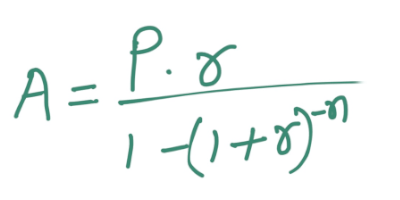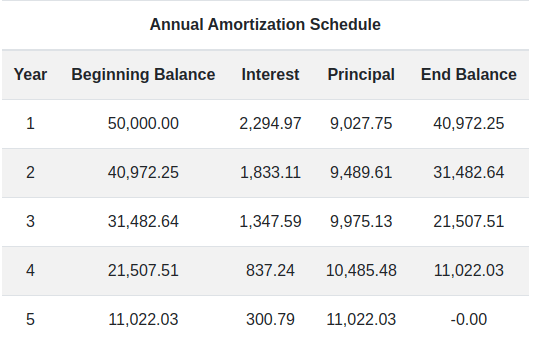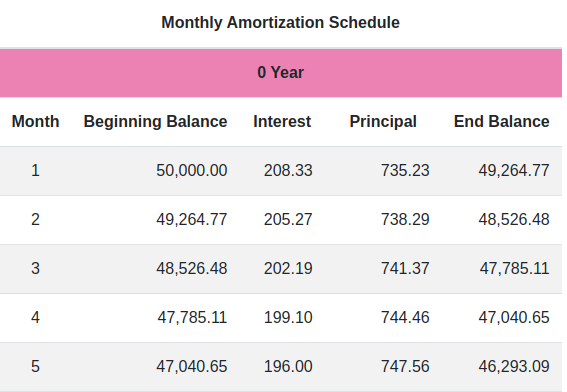# Amortization Calculator

Calculate Loan Payment Schedule

Loan Amount
Year
Interest Rate

## What is Amortization

Amortization is an accounting technique which involves the process of repaying a loan over a time in regular installments, which consist of both interests and principals. Initially, the borrower pays a high interest and least principal but over time, the borrower pays a decreasing amount of interest and an increasing amount of principal to the lender. This process continues until the loan is fully paid off.

## Amortization Calculation Formula

The formula for amortization is:

A = (P * r) / (1 - (1 + r)-n)where:

• A = the payment amount per period or monthly amortization
• r = the monthly interest rate in the form of decimal
• P = the initial principal or loan amount
• n = the total number of payments

### An example of calculation of amortization using this formula :

Let's consider you took the loan of \$50,000 with annual interest of 5%, to be paid completely at the end of 5 years. Here's the value that you want to populate into the formula of amortization mentioned above.

The monthly interest rate would be (r): r = 0.05/12 = 0.0041667 (the percentage is written here in decimal form)

The total number of payments (n) is multiplied with 12, so here n is: n = 5 years x 12 months per year = 60 months (i.e, 60 payments)

Enter the values for r, P, and n into the formula to solve for the monthly payment amount or amortization value (A):

A = (0.0041667 * \$50,000) / (1 - (1 + 0.0041667)^(-60))

A = \$943.56

This gives the result as \$943.56, Which is going to be your monthly loan payment.

Further, to calculate the portion of each payment that you need to pay as interest and principal, you would need the outstanding balance at the beginning of each payment period. For example, the beginning outstanding balance would be \$50,000. So, the interest portion of the payment would be calculated as:

interest = \$50,000 * 0.0041667 = \$208.33

The part of the payment that is paid towards the principal would be:

principal = \$943.56 - \$208.33 = \$735.23

Next, the outstanding balance for the second month would be calculated as:

outstanding balance = \$50,000 - \$735.23 = \$49264.77

And so on, for each upcoming month until the loan is completely paid off, as in this case it’s the 60th month.

## What is Amortization Schedule or Table

Your Loan payment consists of partly interest and partly principal for the predetermined period of time until it is completely paid off. While the payment amount remains the same for each period, the interest rate and the principal varies for each period.

When a borrower gets the loan from its lender, he also receives a table which consists of columns with “beginning balance”, “interest”, “principal” and “ending balance”. This table is simply called the “Amortization Schedule”.

With the help of an amortization schedule, the borrower can keep track of the date of loan payment, amount due , interest due, principal due, and the remaining balance of the loan after each payment. As payments are made on a loan according to its amortization schedule, the proportion of each payment allocated towards interest decreases gradually, while the proportion allocated towards the principal amount increases

The table that shows yearly loan payment is termed as “Yearly Amortization Schedule”, whereas, the table with monthly down payments is called as “Monthly Amortization Schedule”

In the picture below you can see the example of amortization schedule for monthly and yearly loan payments.This amortization calculator is a free online financial tool to calculate amortization online. It lets you find out what amount goes for interest and principal as loan payoff over monthly as well as annually. In comparison to other amortization calculators, it is easier to use and way better than using Excel sheets. It brings you accurate and detailed information in a blink.

As you input the required value like loan amount, term year and interest rate, it takes you to the new page that provides all the repayment information along with charts and tables. The chart consists of graphical representations of the amount paid, interest for each month, and remaining balances. The table includes rows for each payment with columns that show the starting balance, interest rate, principal and the remaining balance. Borrower can take the help of this calculator, to compare and decide how much loan and for what time is going to be suitable for him.

## How to use the Amortization Calculator for loan payment

Well, Calculating amortization on your own can be a daunting task, unless you're using Excel sheets. Even then, filling in the formula and values manually can be a time-consuming task. In such a situation, your best bet would be an online amortization calculator like this one. Not only does it produce results instantly, but it also provides detailed information about the amortization schedule, enabling you to make an informed decision about borrowing beforehand.

Here’s the step by step process to use this calculator.

Step 1 : First you must fill in the loan amount (P) in the input field.

Step 2: Enter the year (n) in which you want to completely pay off the loan

Step 3 : At last enter the interest rate (r) at which you're taking the loan.

Step 4: Click on the 'calculate' button. This will generate a detailed result of your monthly payments and the total cost of lending.